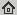# Unifying abstract inexact convergence theorems for descent methods and block coordinate variable metric iPiano

Technical Report , arXiv:1602.07283 [math.OC], 2016
Abstract: An abstract convergence theorem for a class of descent method that explicitly models relative errors is proved. The convergence theorem generalizes and unifies several recent abstract convergence theorems, and is applicable to possibly non-smooth and non-convex lower semi-continuous functions that satisfy the Kurdyka-Lojasiewicz inequality, which comprises a huge class of problems. The descent property is measured with respect to a function that is allowed to change along the iterations, which makes block coordinate and variable metric methods amenable to the abstract convergence theorem. As a particular algorithm, the convergence of a block coordinate variable metric version of iPiano (an inertial forward-backward splitting algorithm) is proved. The newly introduced algorithms perform favorable on an inpainting problem with a Mumford-Shah-like regularization from image processing.

## BibTex reference

```@TechReport{Och16b,
author       = "P. Ochs",
title        = "Unifying abstract inexact convergence theorems for descent methods and block coordinate variable metric iPiano",
institution  = "arXiv:1602.07283 [math.OC]",
month        = " ",
year         = "2016",
url          = "http://lmb.informatik.uni-freiburg.de/Publications/2016/Och16b"
}
```